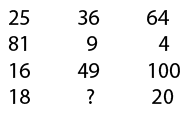### SSC CPO 20 March 2016 Afternoon Shift Question 2

Instructions

In each of the following questions, select the missing number from the given responses.

Question 2

#Solution

The pattern followed here is,

$$\Rightarrow \sqrt{25} + \sqrt{9} + \sqrt{16} = 5 + 3 + 4 = 18$$(vertically)

$$\Rightarrow \sqrt{36} + \sqrt{9} + \sqrt{49} = 6 + 3 + 7 = 16$$(vertically)

$$\Rightarrow \sqrt{64} + \sqrt{4} + \sqrt{100} = 8 + 2 + 10 = 20$$(vertically)

Hence, option C is the correct answer.